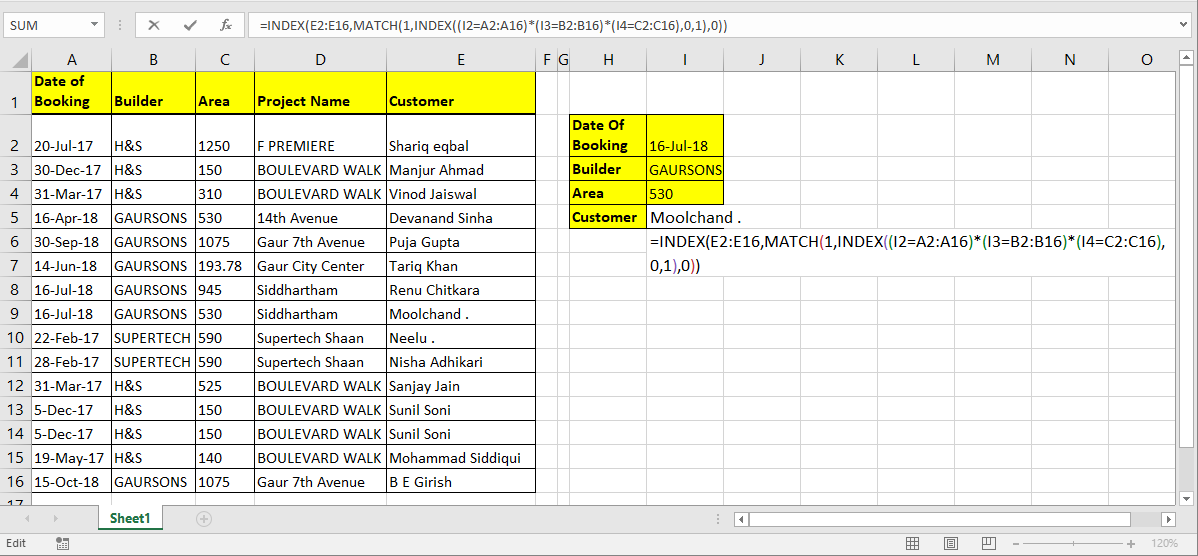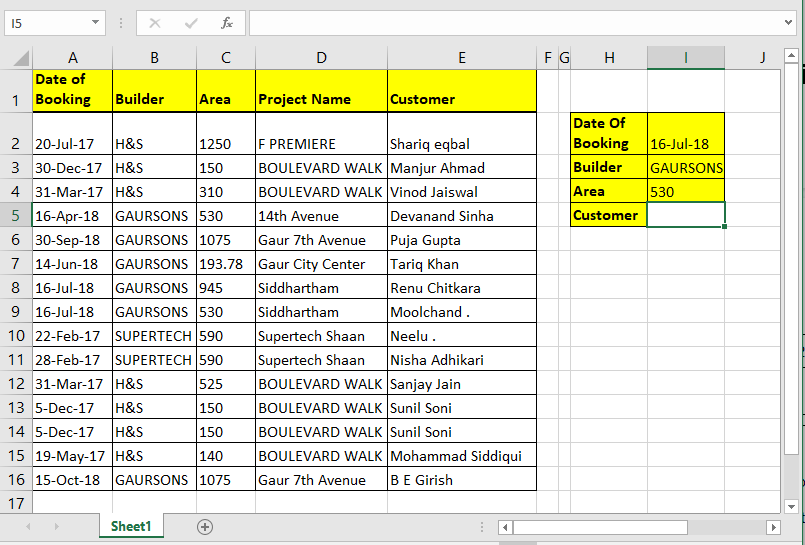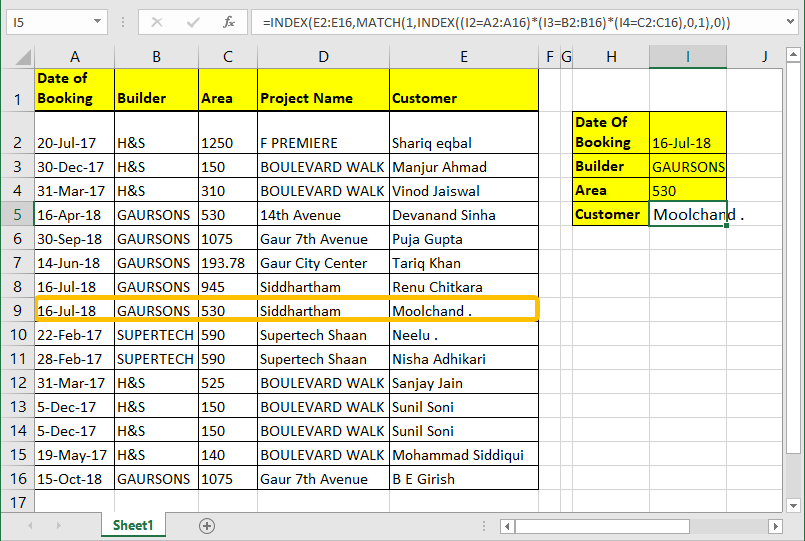How to Get Multiple Values from Same Criteria in Microsoft Excel 2010

In this article, we will learn How to Get Multiple Values from Same Criteria in Microsoft Excel 2010.

Its easy to lookup for a value with one unique key in a table. We can simply use VLOOKUP. But when you don’t have that unique column in your data and need to lookup in multiple columns to match a value, VLOOKUP doesn’t help.

So to lookup a value in a table with multiple criteria we will use INDEX-MATCH-INDEX formula.Generic Formula for Multiple Criteria Lookup

 =INDEX(lookup_range,MATCH(1,INDEX((criteria1 =range1)*(criteria2=range2)*(criteriaN=rangeN),0,1),0))

lookup_range: Its the range from which you want to retrieve value.

Criteria1, Criteria2, Criteria N: These are the criteria you want to match in range1, range2 and Range N. You can have upto 270 criteria - range pairs.

Range1, range2, rangeN : These are the ranges in which you will match your respective criteria.

How it will work? Lets see…

INDEX and MATCH with Multiple Criteria Example

Here I have a data table. I want to pull Name of customer using Date of Booking, Builder and Area. So here I have three criteria and one lookup range.Write this formula in cell I4 hit enter.

 =INDEX(E2:E16,MATCH(1,INDEX((I1=A2:A16)*(I2=B2:B16)*(I3=C2:C16),0,1),0))How it works:

We already know how INDEX and MATCH function work in EXCEL, so i am not going to explain that here. We will talk about the trick we used here.

(I1=A2:A16)*(I2=B2:B16)*(I3=C2:C16): The main part is this. Each part of this statement return an array of true false.

When boolean values are multiplied they return array of 0 and 1. Multiplication works as AND operator. Hense when all value are true only then it returns 1 else 0

(I1=A2:A16)*(I2=B2:B16)*(I3=C2:C16) This altogether will return

 {FALSE;FALSE;FALSE;FALSE;FALSE;FALSE;TRUE;TRUE;FALSE;FALSE;FALSE;FALSE;FALSE;FALSE;FALSE}* {FALSE;FALSE;FALSE;TRUE;TRUE;TRUE;TRUE;TRUE;FALSE;FALSE;FALSE;FALSE;FALSE;FALSE;TRUE}* {FALSE;FALSE;FALSE;TRUE;FALSE;FALSE;FALSE;TRUE;FALSE;FALSE;FALSE;FALSE;FALSE;FALSE;FALSE}

Which will translate into

 {0;0;0;0;0;0;0;1;0;0;0;0;0;0;0}

INDEX((I1=A2:A16)*(I2=B2:B16)*(I3=C2:C16),0,1): INDEX Function will return the same array ({0;0;0;0;0;0;0;1;0;0;0;0;0;0;0}) to MATCH function as lookup array.

MATCH(1,INDEX((I1=A2:A16)*(I2=B2:B16)*(I3=C2:C16),0,1): MATCH function will look for 1 in array  {0;0;0;0;0;0;0;1;0;0;0;0;0;0;0}. And will return index number of first 1 found in array. Which is 8 here.

INDEX(E2:E16,MATCH(1,INDEX((I1=A2:A16)*(I2=B2:B16)*(I3=C2:C16),0,1),0)): Finally, INDEX will return value from given range (E2:E16) at found index (8).

Simple?. Sorry, couldn’t make it more simpler.

Array Solution

If you can hit CTRL + SHIFT + ENTER consequently then you can eliminate the inner INDEX function. Just write this formula and hit CTRL + SHIFT + ENTER.

 =INDEX(E2:E16,MATCH(1,(I1=A2:A16)*(I2=B2:B16)*(I3=C2:C16),0))

Generic Array Formula for Multiple Criteria Lookup

 =INDEX(lookup_range,MATCH(1,(criteria1 =range1)*(criteria2=range2)*(criteriaN=rangeN),0))

Formula works same as above explanation.

Hope this article about How to Get Multiple Values from Same Criteria in Microsoft Excel 2010 is explanatory. Find more articles on extracting values and related Excel formulas here. If you liked our blogs, share it with your friends on Facebook. And also you can follow us on Twitter and Facebook. We would love to hear from you, do let us know how we can improve, complement or innovate our work and make it better for you. Write to us at info@exceltip.com.

Related Articles:

Use VLOOKUP from Two or More Lookup Tables | To lookup from multiple tables we can take an IFERROR approach. To lookup from multiple tables, it takes the error as a switch for the next table. Another method can be an If approach.

How to do Case Sensitive Lookup in Excel | the excel's VLOOKUP function isn’t case sensitive and it will return the first matched value from the list. INDEX-MATCH is no exception but it can be modified to make it case sensitive. Let’s see how…

Lookup Frequently Appearing Text with Criteria in Excel | The lookup most frequently appears in text in a range we use the INDEX-MATCH with MODE function. Here's the method.

Popular Articles :

How to use the IF Function in Excel : The IF statement in Excel checks the condition and returns a specific value if the condition is TRUE or returns another specific value if FALSE.

How to use the VLOOKUP Function in Excel : This is one of the most used and popular functions of excel that is used to lookup value from different ranges and sheets.

How to Use SUMIF Function in Excel : This is another dashboard essential function. This helps you sum up values on specific conditions.

How to use the COUNTIF Function in Excel : Count values with conditions using this amazing function. You don't need to filter your data to count specific values. Countif function is essential to prepare your dashboard.

Terms and Conditions of use

The applications/code on this site are distributed as is and without warranties or liability. In no event shall the owner of the copyrights, or the authors of the applications/code be liable for any loss of profit, any problems or any damage resulting from the use or evaluation of the applications/code.